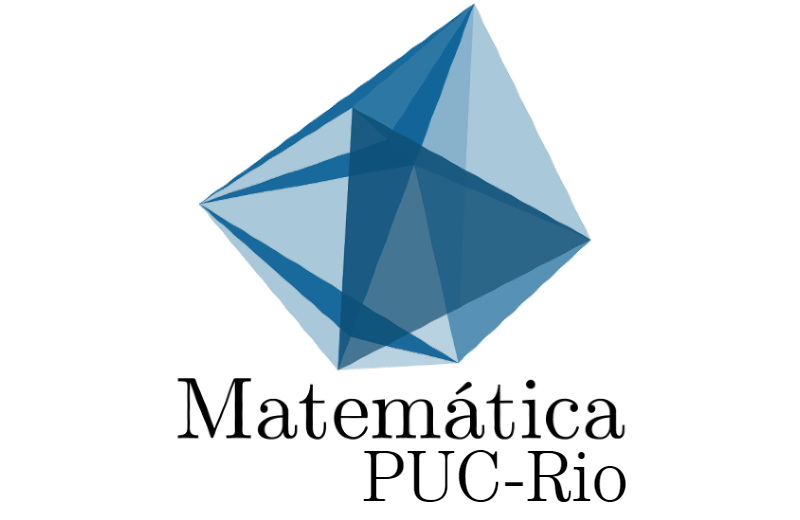SEMINÁRIO SIMPLÉTICO - Gamma conjectures for monotone symplectic manifoldsGamma conjectures for monotone symplectic manifoldsExpositor: Sergey GalkinInstituição: PUC RioData e Horário: 13/09/19 | 16:00h

RESUMO: Gamma conjectures in a computable way relate quantum cohomology of monotone symplectic manifolds to the so-called Gamma class, a characteristic class with coefficients being polynomials in values of Riemann zeta-function. I will explain their formulation and relations to irrationality proofs of Apéry, Dubrovin conjectures and mirror symmetry. The talk is based on joint works with Vasily Golyshev and Hiroshi Iritani (1404.6407, 1404.7388, 1508.00719, 1604.0465